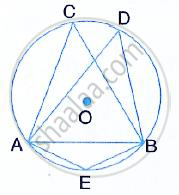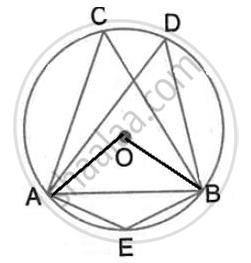Share

# In the Following Figure, O is Centre of the Circle and δAbc is Equilateral. Find: (I) ∠Adb, (Ii) ∠Aeb. - Mathematics

Course

#### Question

In the following figure, O is centre of the circle and ΔABC is equilateral.#### SolutionSince ∠ACBand ∠ADBare in the same segment,
Join OA and OB
Here, ∠AOB =2 ∠ACB = 2×60° = 120°
∠AEB=1/2 Reflex ( ∠AOB) = 1/2 (360°- 120°) 120°
(Angle at the centre is double the angle at the circumference subtended by the same chord)

Is there an error in this question or solution?